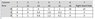# Simplex / Big M method Tableau question

• USN2ENG
In summary, the conversation is about a student seeking help with a maximization problem from a study guide. They are confused about the solution and the use of the a1 column in the tableau. After some discussion, it is determined that the z-row of the tableau is not yet finished and needs to be put into correct form. The only issue with the present tableau is that it has many solutions due to the variable x2.

## Homework Statement

This is from a study guide that I don't have the answers to just yet and I am trying to figure out this last problem for my OR class. I am either confused or the problem is written wrong. I am probably confused though. Any help would be great!

This Tableau is for a Maximization problem. Which of the following cases is true and why?

See the attached screen shot for the Tableau:

A) We have reached a unique optimal solution and the solution is:
B) We have not reached optimal solution. The entering variable is ________ and the exiting variable is ________.
C) The problem is unbounded.
D) There are many optimal solutions because:
E) The problem is infeasible because:

## The Attempt at a Solution

So, this problem confuses me for a couple of reasons. a1 still has M in Row 0, but that could be ok if that is how the math turned out in the row operations. The other problem is that x2 can enter the basis and making it basic will not change z, so we have many solutions. My only problem with that is the problem before this one is this exact Tableau but without the a1 column, so that would be the same answer. So I guess I am expecting that he added the a1 column for a reason and I am not seeing it. Thanks for any help.

#### Attachments

•Simplex.jpg
10.4 KB · Views: 418
USN2ENG said:

## Homework Statement

This is from a study guide that I don't have the answers to just yet and I am trying to figure out this last problem for my OR class. I am either confused or the problem is written wrong. I am probably confused though. Any help would be great!

This Tableau is for a Maximization problem. Which of the following cases is true and why?

See the attached screen shot for the Tableau:

A) We have reached a unique optimal solution and the solution is:
B) We have not reached optimal solution. The entering variable is ________ and the exiting variable is ________.
C) The problem is unbounded.
D) There are many optimal solutions because:
E) The problem is infeasible because:

## The Attempt at a Solution

So, this problem confuses me for a couple of reasons. a1 still has M in Row 0, but that could be ok if that is how the math turned out in the row operations. The other problem is that x2 can enter the basis and making it basic will not change z, so we have many solutions. My only problem with that is the problem before this one is this exact Tableau but without the a1 column, so that would be the same answer. So I guess I am expecting that he added the a1 column for a reason and I am not seeing it. Thanks for any help.

You seem to be forgetting that the tableau is really just a shorthand way of writing the system of equations. In particular, the z-row of the tableau really means
$$z + 0 x_1 + 0x_2 + 5x_3 + 1s_1 + Ma_1 = 15$$
or
$$z = 15 - 5x_3 - s_1 - Ma_1.$$
Having M in the z-row is OK, because a_1 is a nonbasic variable.

Sorry, yeah, that is what I meant when I said it was ok if the math turned out that way with the ERO's. So am I right in assuming that the only thing wrong with this present tableau is that it has many solutions due to x2?